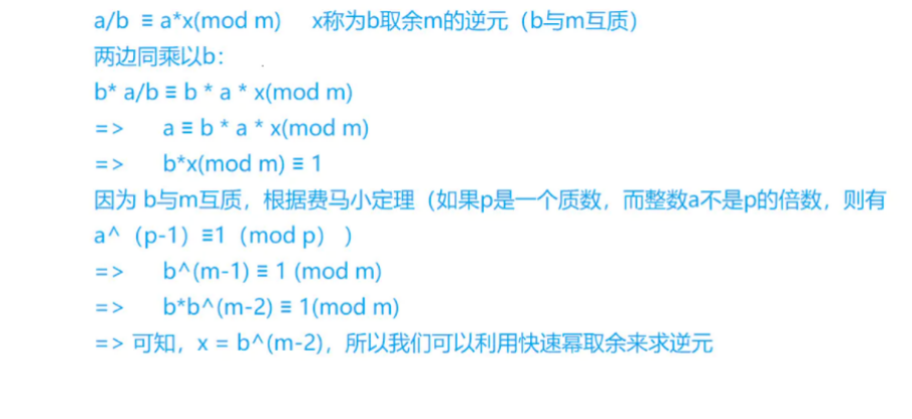# [数论](4)快速幂

## 快速幂

C++ 求a的k次方模p 当 k 不为 0 时 循环 若存在 [ k & 1 == 1] 则 [ res = res * a % p] k右移一位 a平方一次



C++
LL q_pow(LL a,LL k,LL p){
LL res=1;
while(k){
if(k&1) res=res*a%p;//判断这位上是否为1
k>>=1;
a=a*a%p;//a的2次方的下一位
}
return res;
}`C++

# include

using namespace std; typedef long long LL; int n; LL q_pow(LL a,LL k,LL p){//快速幂模板 LL res=1; while(k){ if(k&1) res = res a % p; k>>=1; a=aa%p; } return res; } int main(){ //给定n个a和p,求乘法逆元x scanf("%d",&n); while(n--){ LL a,p; scanf("%lld %lld",&a,&p); if(a%p==0) printf("impossible\n");//a和p不互质 else printf("%lld\n",q_pow(a,p-2,p));//根据费马小定理[当p为质数时可用快速幂] } return 0; }

## 本文链接：

https://nullcode.fun/142.html
1 + 1 =